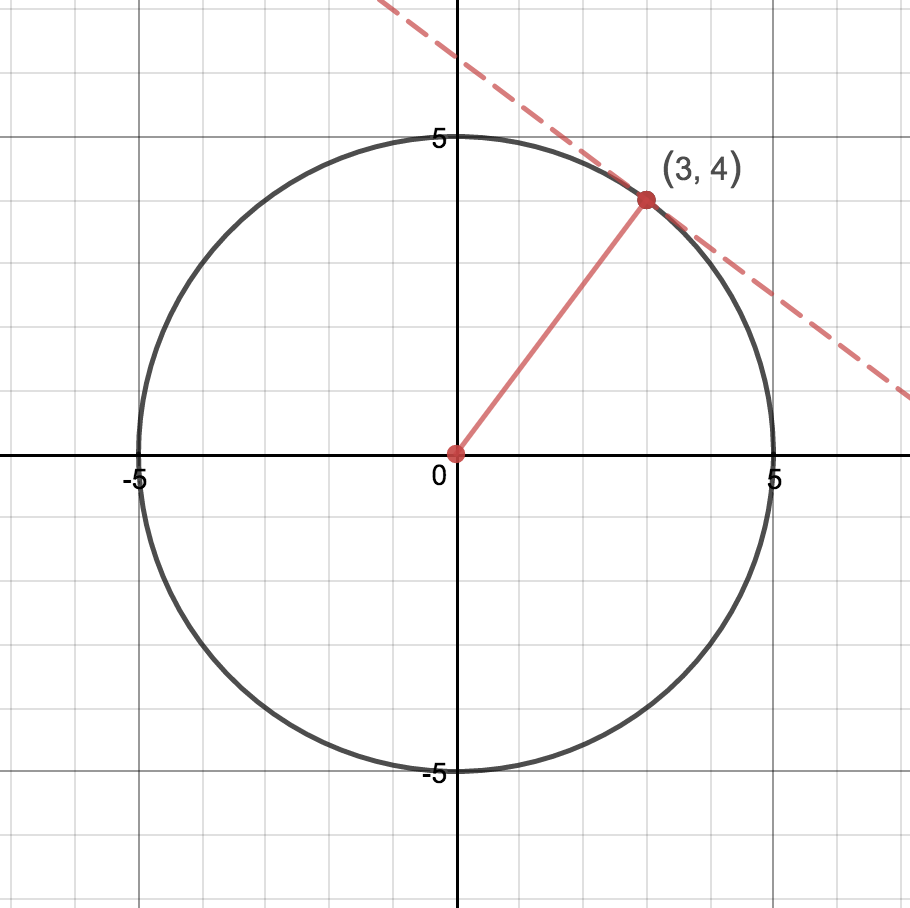### Home > PC3 > Chapter Ch8 > Lesson 8.2.2 > Problem8-79

8-79.

Write the equation of the line tangent to the circle $x^{2} + y^{2} = 25$ at the point $\left(3, 4\right)$.

The radius is perpendicular to the tangent line. Use the diagram at right to determine the slope of the radius and then the slope of the tangent line.

Use the given point and the slope of the tangent line to write the equation.